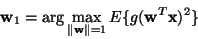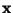Next: Higher-order cumulant tensors Up: Multi-unit contrast functions Previous: Non-linear cross-correlations

### Non-linear PCA criteria

A related approach is offered by the non-linear PCA criteria [82,83,84,112]. These objective functions are based on the idea of introducing a non-linearity in well-known objective functions used in PCA. Take, for example, the recursive definition of PCA in Eq. (3). Introducing a non-linearity g(.) in the formula, one obtains(20)

as a modified principal component of the data. If the non-linearity is suitably chosen as a function of the pdf's of the independent components, and the data is preprocessed by sphering (see Section 5.2), optimizing such non-linear PCA criteria enables estimation of the ICA model. Other definitions of PCA may also be modified by introducing a non-linearity.

Sometimes in connection with the non-linear PCA criteria, a 'bottom-up' approach is used, as with non-linear cross-correlations. Explicit contrast functions may not be formulated, and the algorithms are often obtained directly from existing PCA algorithms by introducing a suitable non-linearity [112,84].

Aapo Hyvarinen
1999-04-23